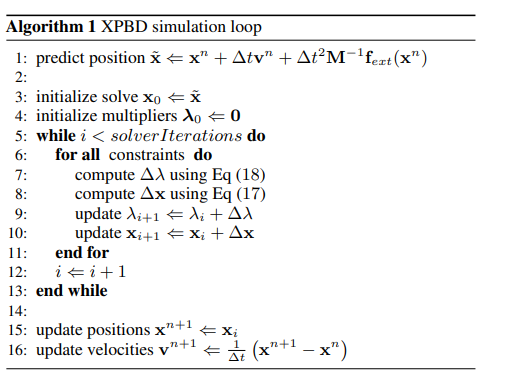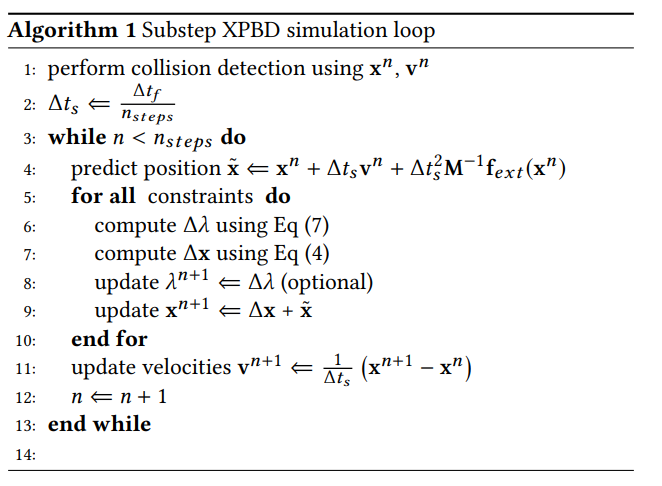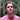# Extended Position Based Dynamics | XPBD

XPBD reduces stability issues and errors that arise in PBD simulations. This week, we'll take a high level look at XPBD to see how it can simplify our simulation loop.

Keywords: position based dynamics, cloth simulation, lagrange, physics, computer graphics

This week I don’t have much time to write a post. That said, I would just like to introduce the topic that we will focus on in the future. This is the subject of XPBD.

XPBD is a response to a stiffness problem encountered with PBD. If we include a stiffness constant, $k$, in the calculation of $\Delta x$ when satisfying constraints, like for example :

$\Delta x = ks\mathbf{M}^{-1}\nabla C(\mathbf{x})$

…it has been found that constraints become infinitely rigid.

More precisely, $k$ is dependent on the time step and the number of iterations of the simulation. The constraints become infinitely rigid as the number of iterations approaches infinity, or as the time step decreases.

Thus, our focus this week is to explore two papers that improve PBD. Here are two articles that can help provide context for today’s discussion:

## XPBD

XPBD aims to eliminate the following dependencies that influence the effective stiffness of the system:

• The time step
• The number of iterations performed when satisfying the constraints.

This problem occurs during simulations where several types of materials are used (i.e. soft bodies interacting with rigid bodies.)

To solve this problem, the PBD algorithm is extended to have a direct correspondence with Newton’s second law.

Regardons l’algorithme proposé :Recall the PBD simulation loop we talked about in my last article. The most important difference is this idea of iteratively calculating $\lambda$ at line (9).

Specifically, the calculation of the change in the Lagrange multiplier, $\Delta \lambda$, during one iteration of the solver is defined as follows:

$\Delta \lambda = \frac{-C(\mathbf{x}) - \tilde{\alpha} \lambda}{\nabla C(\mathbf{x}) \mathbf{M}^{-1} \nabla C(\mathbf{x})^T + \tilde{\alpha}}$

where $\tilde{\alpha}$ is a block diagonal compliance matrix corresponding to the inverse of the stiffness constants, $\frac{1}{k}$. In addition, the constraints are regularized with $\tilde{\alpha}$ which results from the factorization of a quadratic energy potential.

In terms of the time step, we can say that:

$\tilde{\alpha} = \frac{\alpha}{\Delta t^2}$

When $\alpha = 0$, we arrive at the PBD equation! This fact is not shocking if we consider that a compliance factor equal to 0 is similar to a stiffness equal to infinity, $\infty = 1 / 0$.

All that said, we can define $\Delta \mathbf{x}$ as follows:

$\Delta \mathbf{x} = \mathbf{M}^{-1}\nabla C(\mathbf{x})^T\Delta \lambda$

This is all a little hand-wavy, but rest assured that we will deal with the derivation of all of these new equations in a later article.

## Small Steps

As it turns out, we can make this simulation even better. By taking smaller time steps to advance the simulation, rather than iteratively solving the system of constraints for each larger time step, we can increase stability of the system and reduce error for a wide range of stiffness parameters:What’s interesting is that we have no reason to keep storing $\lambda$ because we are solving the constrained functions only once for each small step. In other words, the modification of $\lambda$ does not propagate to future constraint solving iterations, which is contrary to PBD.

Since we only perform one iteration per time substep and the initial value of the Lagrange multiplier is 0, we can rewrite the equation $\Delta \lambda$ as follows:

$\Delta \lambda = \frac{-C(\mathbf{x})}{\nabla C(\mathbf{x}) \mathbf{M}^{-1} \nabla C(\mathbf{x})^T + \tilde{\alpha}}$

This equation will be used during the cloth simulation!

As I already mentioned, this week our conversation would be short because I have a busy week ahead of me! In a later article, we will see the derivations of all the equations we have just seen.

## Comments for Extended Position Based Dynamics | XPBDWritten by Carmen Cincotti, computer graphics enthusiast, language learner, and improv actor currently living in San Francisco, CA.  Follow @CarmenCincotti